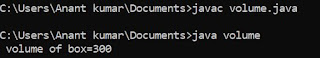## Friday, 29 June 2018

### Difference between Call by value and Call by references using program of swapping of variable in C

Using call by value method :
#include <stdio.h>
void swap ( int , int ) ;
void main ()
{
int a , b , ;
clrscr () ;
printf ("\n Enter value of a:") ;
scanf ("%d", & a) ;
printf ("\n Enter value of b:") ;
scanf ("%d", & b) ;
swap ( a , b ) ;
getch () ;
}
void swap ( int a , int b )
{
int temp ;
temp = a ;?
a = b ;
b = temp ;
printf ("\n %d", a) ;
printf ("\n %d", b) ;
}

Working :   In this program the value of actual argument will be copied in the formal argument which is written in main () and function definition respectively .
These two types of argument will be totally different because of different address the changes which is done in the function definition will not be visible in the main function . This method in which the value of main function is copied in the variables of function definition is known as the call by value method or argument passed by value method .

Using call by references method :

/* swapping of variables */
#include <stdio.h>
void swap ( int* , int * ) ;
void main ()
{
int a ,b ;
clrscr () ;
printf ("\n Enter a number:") ;
scanf ("%d", &a) ;
printf ("\n Enter a number:") ;
scanf ("%d", &b) ;
swap ( &a , &b ) ;
printf ("\n a = %d b = %d", a,b) ;
getch () ;
}
void swap ( int *a , int *b )
{
int temp ;
temp = *a ;
*a = *b ;
*b = temp ;
}

Working :   In these types of program if we pass the address from main function to the function definition then these address must be stored in a special types of variable and the changes will be visible throughout the program in the function definition and as well as the main function .